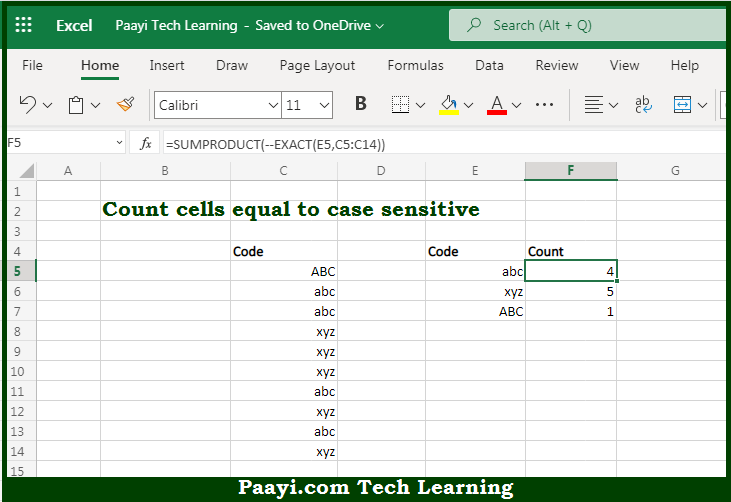# Learn How to Count Cells Equal To Case Sensitive In Microsoft Excel

Written by | 0 Comments | 597 Views

In this article, you will learn how to use the Microsoft Excel COUNT formula and its purpose in Microsoft Excel. You will also get to know how to count cells equal to case sensitive and see the generic formula.

Count Cells Equal To Case Sensitive Using Microsoft Excel Count Formula

The main purpose of the Microsoft Excel COUNT formula is to count cells that contain specific text. Here we will learn how to count cells that are case sensitive.  That implies, with the help of the COUNT formula you can able to count cells that contain specific text, taking into account upper and lower case, you can use a formula based on the EXACT function together with the SUMPRODUCT function. So, with the help of the COUNT formula, you can able to count cells that contain specific text which is case sensitive.

General Syntax to Count Cells Equal To Case Sensitive

=SUMPRODUCT(--EXACT(value,range))

The Explanation for the Count Cells Equal To Case SensitiveSo we know that Microsoft Excel COUNT formula you can able to count cells that contain specific text. Here we will learn how to count cells that are case sensitive. Our main aim is to count codes in a case-sensitive way. You can use both COUNTIF and COUNTIFS functions for counting text values, but neither is case-sensitive, so they can't be used to solve this problem.

It should be noted that SUMPRODUCT can handle arrays natively, so it is not required to use Control+Shift+Enter to enter this formula. So, with the help of the COUNT formula, you can able to count cells that contain specific text.